## Photon Absorption Coefficient:

Photon Absorption Coefficient – When light falls on a semiconductor, the photons may be absorbed or they may propagate through the semiconductor depending on the photon energy (E) and the band gap energy EG. Where the photon energy is less than EG, the photons are not readily absorbed and the light is transmitted through the material and the semiconductor appears to be transparent.

The probability of interaction between the photons and a valence electron is high whenever E = hf > EG. This interaction generates an electron in the conduction band and a hole in the valence band—known as elec­tron-hole pair (EHP). The excess energy provides the electron or hole additional kinetic energy which heats the semiconductor.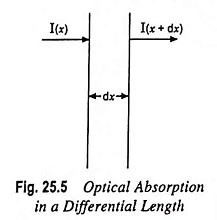The intensity of photon flux I(x) is expressed in terms of en­ergy/cm2-s. Fig. 25.5 depicts an incident photon intensity at a po­sition x and the photon flux emerging at a distance x + dx. The energy absorbed per unit time in the distance dx is given by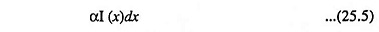where α is the photon absorption coefficient (the relative number of photons absorbed per unit distance, given in units of cm-1)

From Fig. 25.5, we may write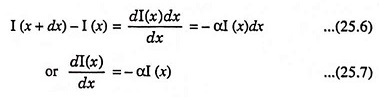From initial condition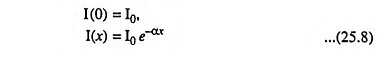If It be the intensity of transmitted beam after traversing the sample thickness t, thenThe intensity of photon flux falls exponentially with distance through the semiconductor material as illustrated in Fig. 25.6. If the photon absorption coefficient is large, the photons are absorbed over a relatively short distance.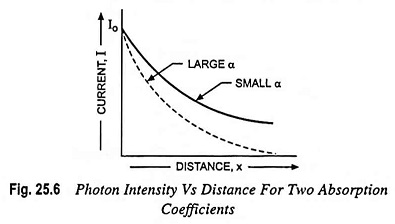The absorption coefficient in the semiconductor is a very strong function of photon energy and band gap energy. This coefficient varies with the photon wavelength and with material.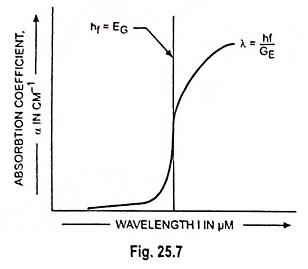In a typical plot of absorption coefficient α versus wavelength, depicted in Fig. 25.7, there is negligible absorption at long wavelengths (hf small) and considerable absorption of photons with energies larger than EG. According to Eq. (25.4) the relation between photon energy and wavelength is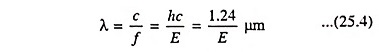Scroll to Top